Screenshots

•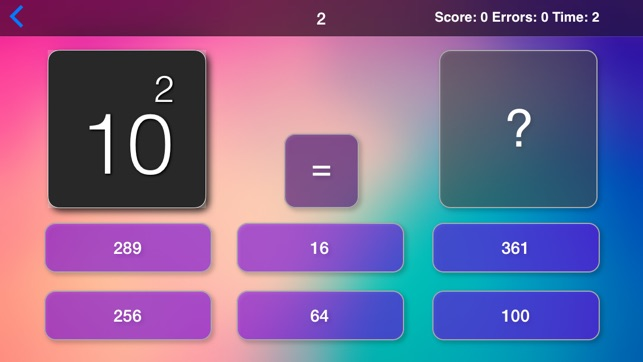•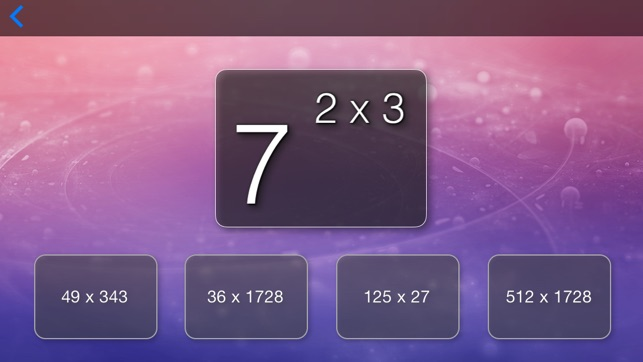•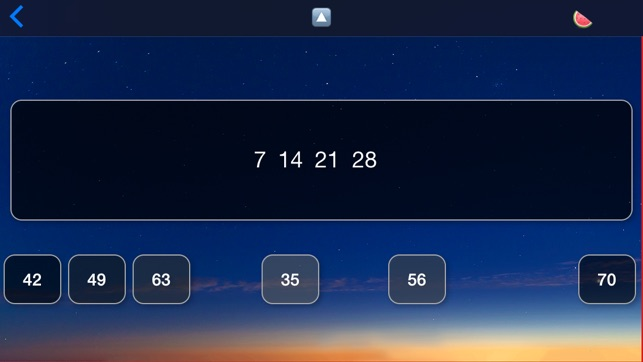•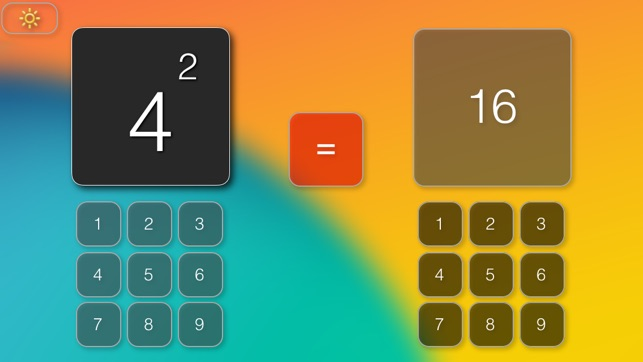•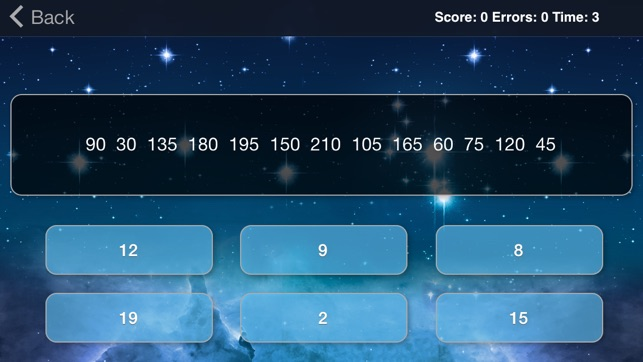Description

Yolaroo Numbers is a great mathematical learning app.

Learn or teach exponents, numbers, series, and sequences, quickly and easily in a simple but advanced new way.

Yolaroo Numbers has 10 different activities.

- The exponential computation activity teaches or tests students on their knowledge of exponents. The student can practice solving squares, cubes, and numbers up to numbers to the 6th power.

- The exponential Numberator activity introduces exponents. The student can discover how the relationships between these numbers are expressed.

- The exponential number activity lets students discover exponential number sequences in an interactive way. The student can explore squared numbers or the roots of numbers across the series of integers: n^2, n^1/2, etc.

- The four exponential math activities teach addition, subtraction, and multiplication of exponents.

- The series activity teaches students how to identify the base multiple of an out-of-order series.
{8 6 10 2 4} -> 2n: {2 4 6 8 10}

- The series order activity teaches students how to put an out-of-order series into the correct order (either from the last number or the first number in the sequence).
{10 5 15 25 20} -> {5 10 15 20 25} or {25 20 15 10 5}

- The series identification activity lets students discover different number sequences in an interactive way through base multiples: 2n, 10n, etc.

Everyone needs a good education. This app is 100% free, because learning and education are important for everyone.

Version 2.2

fixed a typo

Ratings and Reviews

3.3 out of 5
3 Ratings

3 Ratings

Game winner 2566854330754 ,

Works

This really works

Math really ,

Stupid

This is the most ignorantly made app every

Information

Seller
Matthew Moscatello
Size
27.8 MB
Category
Education
Compatibility

Requires iOS 7.0 or later. Compatible with iPhone, iPad, and iPod touch.

Languages

English

Age Rating
Rated 4+
Price
Free

Supports

•